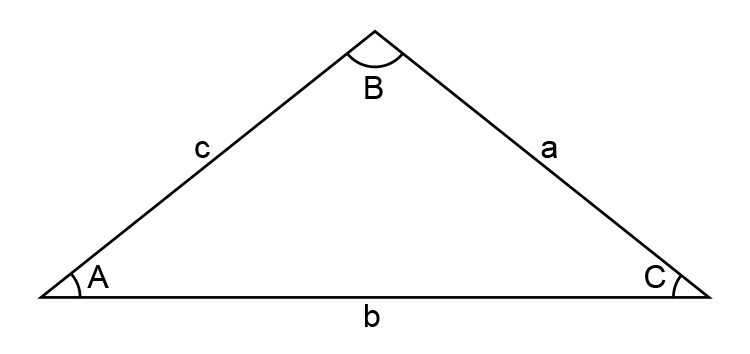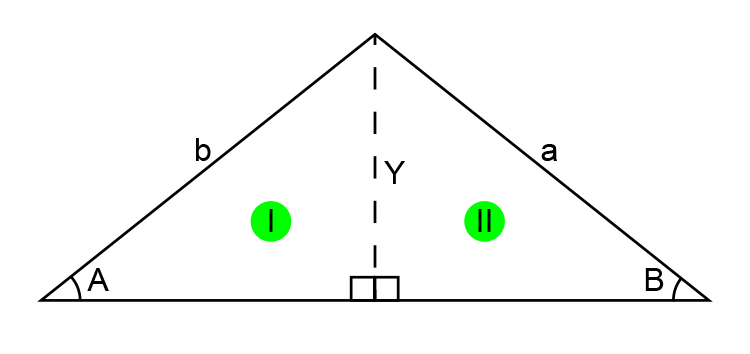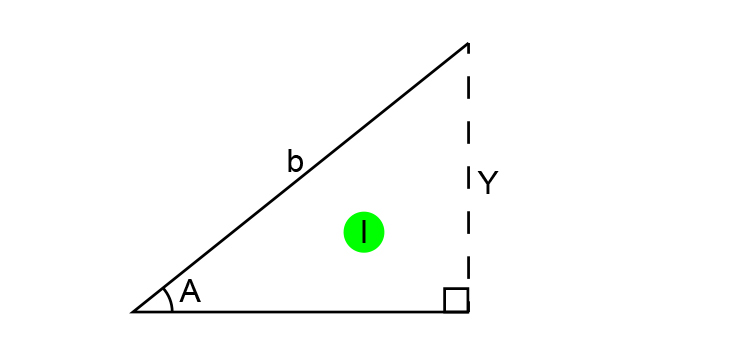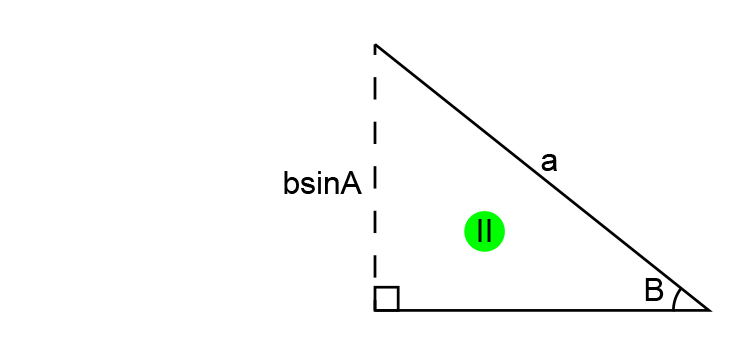# Where does the sine rule come from?

For the mathematical puritans out there the sine rule derives from the following:

(you really don’t need to know this)Find “a” KNOWING the angle A and length b and c.

Redraw the diagramFind Y in the triangle IUse trigonometry which tells us that sin\ theta=(O)/H

sinA=Y/b

Therefore: Y=bsinA

Find “a” in triangle IIUse trigonometry which tells us that sin\ theta=(O)/H

sinB=(bsinA)/a

Therefore  a=(bsinA)/sinB

Or  a/sinA=b/sinB  (the sine rule)

But again we emphasise that you really don’t need this.